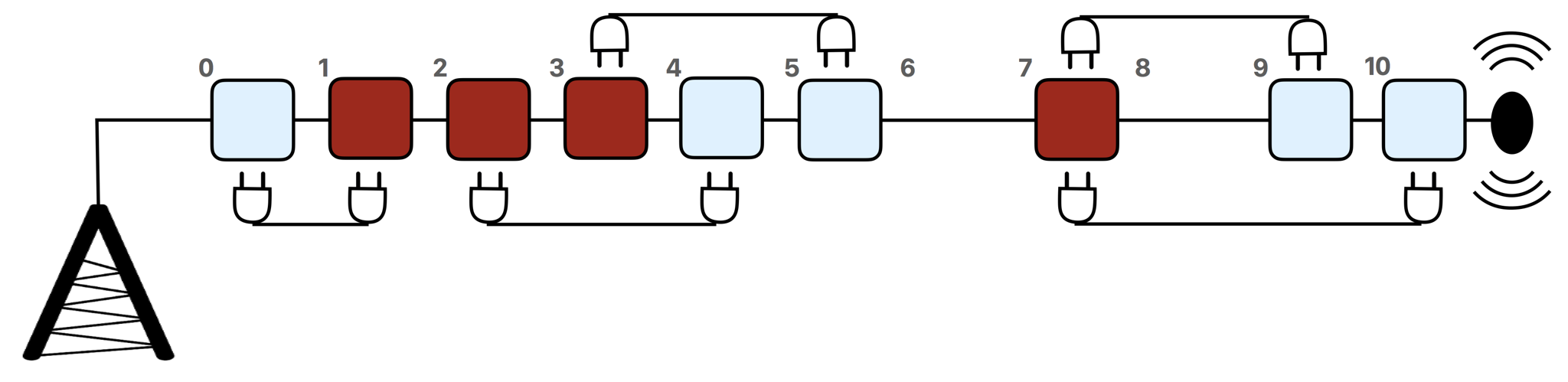시간 제한 메모리 제한 제출 정답 맞은 사람 정답 비율
1 초 512 MB 43 27 25 65.789%

## 문제

Maryam is an electrical engineer. She is designing wiring on a communication tower. On the tower there are some connection points, placed at distinct heights. A wire can be used to connect any two connection points. Each connection point can be connected to an arbitrary number of wires. There are two types of connection points: red and blue.

For the purpose of this problem the tower should be viewed as a line and the connection points as blue and red points that are at non-negative integer coordinates on this line. The length of a wire is the distance between the two connection points it connects.

Your goal is to help Maryam find a wiring scheme such that:

1. Each connection point has at least one wire to a connection point of a different color.
2. The total length of the wires is minimized.

## 구현

You should implement the following procedure:

int64 min_total_length(int[] r, int[] b)

• $r$: array of length $n$ containing the positions of the red connection points in increasing order.
• $b$: array of length $m$ containing the positions of the blue connection points in increasing order.
• This procedure should return the minimum total length of wires, among all valid wiring schemes.
• Note that the return type of this procedure is int64.

## 예시

min_total_length([1, 2, 3, 7], [0, 4, 5, 9, 10])


The figure below illustrates this example.• The tower is shown horizontally.
• In the black-and-white printed version of the problem statement the red connection points are dark and the blue ones are light.
• There are $4$ red connection points, located at positions $1, 2, 3,$ and $7$.
• There are $5$ blue connection points, located at positions $0, 4, 5, 9,$ and $10$.
• One optimal solution is shown in the figure above.
• In this solution, the total length of the wires is $1 + 2 + 2 + 2 + 3 = 10$, which is optimal. So, the procedure should return $10$.
• Note that two wires are connected to the connection point at position $7$.

## 제한

• $1 \leq n, m \leq 100\,000$,
• $0 \leq r[i] \leq 10^9$ (for all $0 \leq i \leq n-1$),
• $0 \leq b[i] \leq 10^9$ (for all $0 \leq i \leq m-1$),
• Each of the arrays $r$ and $b$ is sorted in ascending order.
• All $n+m$ values in the arrays $r$ and $b$ are distinct.

## 서브태스크

번호 배점 제한
1 7

$n, m \leq 200$

2 13

All red connection points have positions smaller than any blue connection points.

3 10

There is at least one red connection point and one blue connection point among every $7$ consecutive connection points.

4 25

All connection points have different positions in the range $[1, n+m]$.

5 45

No additional constraints.

## 샘플 그레이더

The sample grader reads the input in the following format:

• line $1$: $\;\; n \;\; m$
• line $2$: $\;\; r \;\; r \; \ldots \; r[n-1]$
• line $3$: $\;\; b \;\; b \; \ldots \; b[m-1]$

The sample grader prints a single line containing the return value of min_total_length.

## 제출할 수 있는 언어

C++17, C++14, C++20, C++14 (Clang), C++17 (Clang), C++20 (Clang)

## 채점 및 기타 정보

• 예제는 채점하지 않는다.